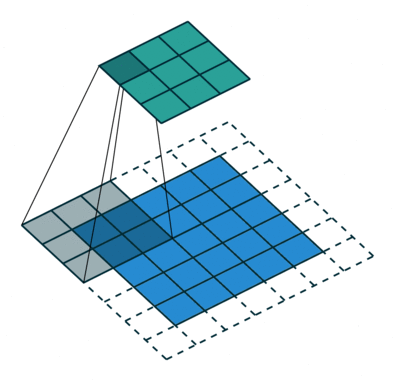# Complex Convolution¶

## Complex Conv 2D¶

Small code example

input_shape = (4, 28, 28, 3)
x = tf.cast(tf.random.normal(input_shape), tf.complex64)
y = ComplexConv2D(2, 3, activation='cart_relu', padding="same", input_shape=input_shape[1:], dtype=x.dtype)(x)
assert y.shape == (4, 28, 28, 2)
assert y.dtype == tf.complex64

class ComplexConv2D

2D convolution layer (e.g. spatial convolution over images). Support complex and real input.This layer creates a convolution kernel that is convolved with the layer input to produce a tensor of outputs. If use_bias is True, a bias vector is created and added to the outputs. Finally, if activation is not None, it is applied to the outputs as well. When using this layer as the first layer in a model, provide the keyword argument input_shape (tuple of integers, does not include the sample axis), e.g. input_shape=(128, 128, 3) for 128x128 RGB pictures in data_format="channels_last".

__init__(self, filters, kernel_size, strides=(1, 1), padding='valid', data_format=None, dilation_rate=(1, 1), groups=1, activation=None, use_bias=True, dtype=np.complex64, kernel_initializer=ComplexGlorotUniform(), bias_initializer=Zeros(), kernel_regularizer=None, bias_regularizer=None, activity_regularizer=None, kernel_constraint=None, bias_constraint=None, init_technique: str = 'mirror', **kwargs)
Parameters: filters – Integer, the dimensionality of the output space (i.e. the number of output filters in the convolution). kernel_size – An integer or tuple/list of 2 integers, specifying the height and width of the 2D convolution window. Can be a single integer to specify the same value for all spatial dimensions. strides – An integer or tuple/list of 2 integers, specifying the strides of the convolution along the height and width. Can be a single integer to specify the same value for all spatial dimensions. Specifying any stride value != 1 is incompatible with specifying any dilation_rate value != 1. padding – one of "valid" or “same” (case-insensitive). - "valid" means no padding. - "same" results in padding evenly to the left/right or up/down of the input such that output has the same height/width dimension as the input. dtype – Dtype of the input and therefore layer. Default complex64. data_format – A string, one of channels_last (default) or channels_first. The ordering of the dimensions in the inputs. channels_last corresponds to inputs with shape (batch_size, height, width, channels) while channels_first corresponds to inputs with shape (batch_size, channels, height, width). It defaults to the image_data_format value found in your Keras config file at ~/.keras/keras.json. If you never set it, then it will be channels_last. dilation_rate – an integer or tuple/list of 2 integers, specifying the dilation rate to use for dilated convolution. Can be a single integer to specify the same value for all spatial dimensions. Currently, specifying any dilation_rate value != 1 is incompatible with specifying any stride value != 1. groups – A positive integer specifying the number of groups in which the input is split along the channel axis. Each group is convolved separately with filters / groups filters. The output is the concatenation of all the groups results along the channel axis. Input channels and filters must both be divisible by groups. activation – Activation function to use. If you don’t specify anything, no activation is applied (see keras.activations or cvnn.activations). For complex dtype, this must be a cvnn.activations module. use_bias – Boolean, whether the layer uses a bias vector. kernel_initializer – Initializer for the kernel weights matrix (see cvnn.initializers). bias_initializer – Initializer for the bias vector (see cvnn.initializers). kernel_regularizer – Regularizer function applied to the kernel weights matrix (see keras.regularizers). bias_regularizer – Regularizer function applied to the bias vector (see keras.regularizers). activity_regularizer – Regularizer function applied to the output of the layer (its “activation”) (see keras.regularizers). kernel_constraint – Constraint function applied to the kernel matrix (see keras.constraints). bias_constraint – Constraint function applied to the bias vector (see keras.constraints). init_technique – String. One of ‘mirror’ or ‘zero_imag’. Tells the initializer how to init complex number if the initializer was tensorflow’s built in initializers (not supporting complex numbers). ’mirror’ (default): Uses the initializer for both real and imaginary part. Note that some initializers such as Glorot or He will lose it’s property if initialized this way. ’zero_imag’: Initializer real part and let imaginary part to zero.

Warning

ATTENTION: regularizers not yet working, that parameter will be ignored.

call(self, inputs)
Calls convolution, this function is divided in 4:
1. Input parser/verification
2. Convolution
3. Bias
4. Activation Function
Parameters: inputs – Input tensor, or list/tuple of input tensors. A tensor of rank 4+ representing activation(conv2d(inputs, kernel) + bias).

## Complex Conv 1D and 3D¶

This library also has 1D (ComplexConv1D) and 3D (ComplexConv3D) convolution layers. Usage is analogous to ComplexConv2D.